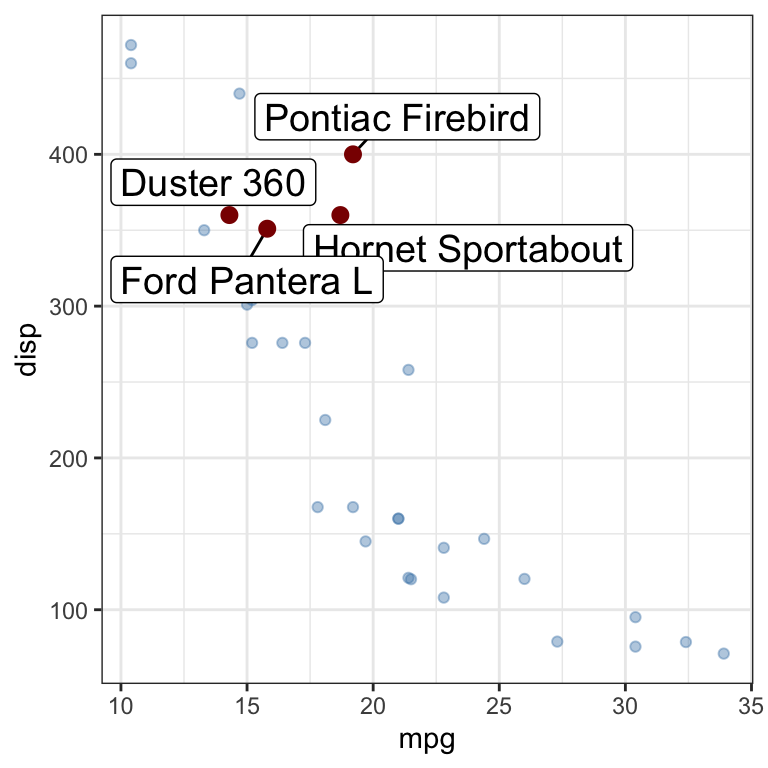# gghighlight: Easy Way to Highlight a GGPlot in R#### gghighlight: Easy Way to Highlight a GGPlot in R

This article presents how to easily highlight a ggplot using the gghighlight package.

Contents:

## Prerequisites

Load required packages and set the default ggplot2 theme to theme_bw().

library(tidyverse)
library(gghighlight)
theme_set(theme_bw())

## Line plot

• Basic line plot
p <- ggplot(
airquality,
aes(Day, Temp, group = Month, color = factor(Month))
) +
geom_line() +
scale_color_viridis_d() +
labs(x = "Day of Month", y = "Temperature") +
theme(legend.position = "top")
p• Highlight the lines whose max values are larger than 93 like below:
p + gghighlight(max(Temp) > 93, label_key = Month)## Histogram

ggplot(iris, aes(Sepal.Length, fill = Species)) +
geom_histogram(bins = 30) +
scale_fill_viridis_d() +
gghighlight() +
facet_wrap(~ Species)## Scatter plot

df <- mtcars %>% mutate(name = row.names(.))
df %>%
ggplot(aes(mpg, disp)) +
geom_point(col = "darkred") +
gghighlight(disp > 350 & disp <= 400,
unhighlighted_colour = alpha("steelblue", 0.4),
use_direct_label = TRUE,
label_key = name,
label_params = list(size = 5)) +
geom_point(col = "darkred", size = 2.5)## Bar plot

ggplot(df, aes(name, mpg)) +
geom_col() +
theme(axis.text.x = element_text(angle = 45, hjust = 1)) +
gghighlight(mpg > 25)•Joseph Sékou B. Dembélé

I would like to realize a boxplot analysis with ggpubr library but I didn’t arrive to do it. In effect I have some questions to address you:
What is the adequate format to export the phenotyping data (excel, csv, test)?
is there a script appropriate to vizualise the phenotyping data for this library?
would he be possible to give the script to someaone?

•Kassambara

Hi,

Here are the steps for creating a box plot:

1. Organize your data as follow:

  group value
1     a    10
2     a    13
3     a    11
4     b    30
5     b    31
6     b    20


2. Prepare your data as described at: Data preparation best practices
3. Save your data into csv format

4. Import the data into R (http://www.sthda.com/english/wiki/reading-data-from-txt-csv-files-r-base-functions):

read.csv(file.choose())


5. Create the box plot:

library(ggpubr)
ggboxplot(mydata, x = "group", y = "value")


If it does not work, send me a sample of your data by e-mail at contact@datanovia.com